## miceRanger: Fast Imputation with Random Forests

`miceRanger` performs Multiple Imputation by Chained Equations (MICE) with random forests. It can impute categorical and numeric data without much setup, and has an array of diagnostic plots available.
This README contains an introduction to multiple imputation, as well as a thorough walkthrough of the package. More information on MICE can be found in Stef van Buuren's excellent online book, which you can find here.

## The MICE Algorithm

Multiple Imputation by Chained Equations 'fills in' (imputes) missing data in a dataset through an iterative series of predictive models. In each iteration, each specified variable in the dataset is imputed using the other variables in the dataset. These iterations should be run until it appears that convergence has been met.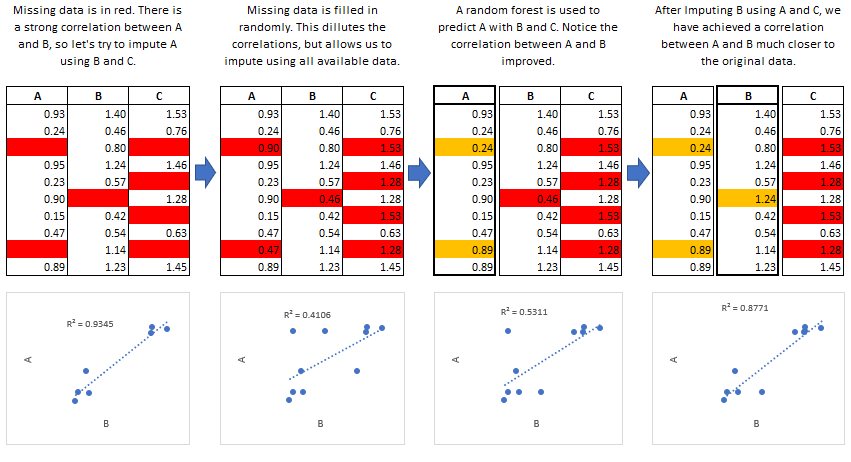This process is continued until all specified variables have been imputed. Additional iterations can be run if it appears that the average imputed values have not converged, although no more than 5 iterations are usually necessary.

### Common Use Cases

##### Data Leakage:

MICE is particularly useful if missing values are associated with the target variable in a way that introduces leakage. For instance, let's say you wanted to model customer retention at the time of sign up. A certain variable is collected at sign up or 1 month after sign up. The absence of that variable is a data leak, since it tells you that the customer did not retain for 1 month.

##### Funnel Analysis:

Information is often collected at different stages of a 'funnel'. MICE can be used to make educated guesses about the characteristics of entities at different points in a funnel.

##### Confidence Intervals:

MICE can be used to impute missing values, however it is important to keep in mind that these imputed values are a prediction. Creating multiple datasets with different imputed values allows you to do two types of inference:

• Imputed Value Distribution: A profile can be built for each imputed value, allowing you to make statements about the likely distribution of that value.
• Model Prediction Distribution: With multiple datasets, you can build multiple models and create a distribution of predictions for each sample. Those samples with imputed values which were not able to be imputed with much confidence would have a larger variance in their predictions.

### Predictive Mean Matching

`miceRanger` uses a procedure called predictive mean matching (PMM) to select which values are imputed. PMM involves selecting a datapoint from the original, nonmissing data which has a predicted value close to the predicted value of the missing sample. The closest N (`meanMatchCandidates` parameter in `miceRanger()`) values are chosen as candidates, from which a value is chosen at random. Going into more detail from our example above, we see how this works in practice: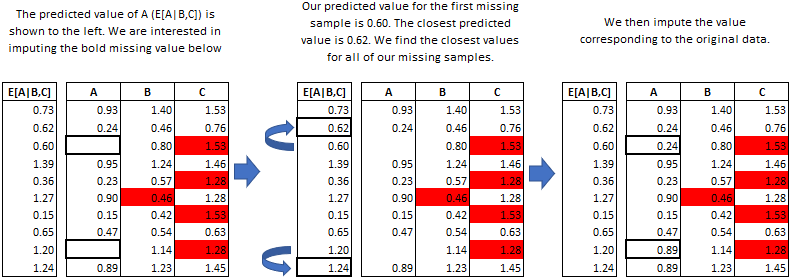## Using miceRanger

In these examples we will be looking at a (contrived) example of multiple imputation. We need to load the packages, and define the data:

``````require(miceRanger)
require(data.table)
set.seed(1)

# Load data
data(iris)
setDT(iris)

# Ampute the data. iris contains no missing values by default.
ampIris <- amputeData(iris,perc=0.25)
head(ampIris,10)
``````
``````##     Sepal.Length Sepal.Width Petal.Length Petal.Width Species
##  1:          5.1         3.5           NA         0.2    <NA>
##  2:          4.9         3.0          1.4         0.2  setosa
##  3:          4.7         3.2          1.3         0.2  setosa
##  4:          4.6         3.1          1.5         0.2  setosa
##  5:          5.0         3.6          1.4         0.2  setosa
##  6:          5.4         3.9          1.7         0.4  setosa
##  7:           NA         3.4          1.4         0.3  setosa
##  8:          5.0         3.4          1.5         0.2  setosa
##  9:          4.4         2.9          1.4         0.2    <NA>
## 10:          4.9         3.1          1.5         0.1  setosa
``````

### Simple example

``````# Perform mice, return 8 datasets.
seqTime <- system.time(
miceObj <- miceRanger(
ampIris
, m=6
, verbose=FALSE
)
)
``````

### Running in Parallel

By default, `ranger` will use all available cores. However, we can still save a lot of time by sending each dataset imputation to a different R back end. To do this, we need to set up some parallel back ends and use `parallel = TRUE`. Fair warning: This causes the dataset to be copied for each back end, which may eat up your RAM.

``````library(doParallel)

# Set up back ends.
cl <- makeCluster(2)
registerDoParallel(cl)

# Perform mice
parTime <- system.time(
miceObjPar <- miceRanger(
ampIris
, m=6
, parallel = TRUE
, verbose = FALSE
)
)
stopCluster(cl)
registerDoSEQ()
``````

Let's take a look at the time we saved running in parallel:

``````perc <- round(1-parTime[]/seqTime[],2)*100
print(paste0("The parallel process ran ",perc,"% faster using 2 R back ends."))
``````
``````##  "The parallel process ran 2% faster using 2 R back ends."
``````

We did not save that much time by running in parallel. `ranger` already makes full use of our CPU. If we were running more datasets or iterations, we would have have seen a greater decrease in time over the sequential process.

### Adding More Iterations/Datasets

If you plot your data and notice that you need to may need to run more iterations, you can do so by simply using the `addIterations()` function.

``````miceObj <- addIterations(miceObj,iters=2,verbose=FALSE)
miceObj <- addDatasets(miceObj,datasets=1,verbose=FALSE)
``````

## Diagnostic Plotting

`miceRanger` comes with an array of diagnostic plots that tell you how valid the imputations may be, how they are distributed, which variables were used to impute other variables, and so on.

### Distribution of Imputed Values

We can take a look at the imputed distributions compared to the original distribution for each variable:

``````plotDistributions(miceObj,vars='allNumeric')
``````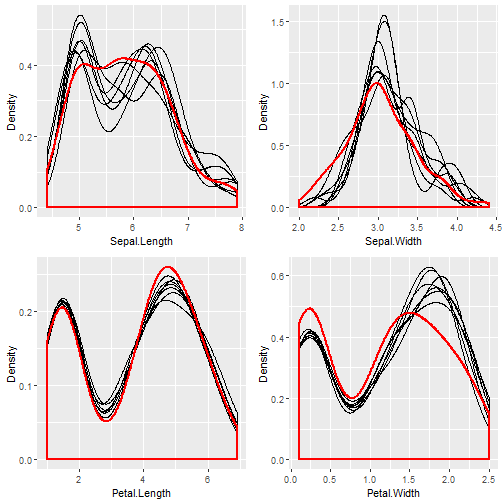The red line is the density of the original, nonmissing data. The smaller, black lines are the density of the imputed values in each of the datasets. If these don't match up, it's not a problem, however it may tell you that your data was not Missing Completely at Random (MCAR).

### Convergence of Correlation

We are probably interested in knowing how our values between datasets converged over the iterations. The `plotCorrelations` function shows you a boxplot of the correlations between every combination of datasets, at each iteration:

``````plotCorrelations(miceObj,vars='allNumeric')
``````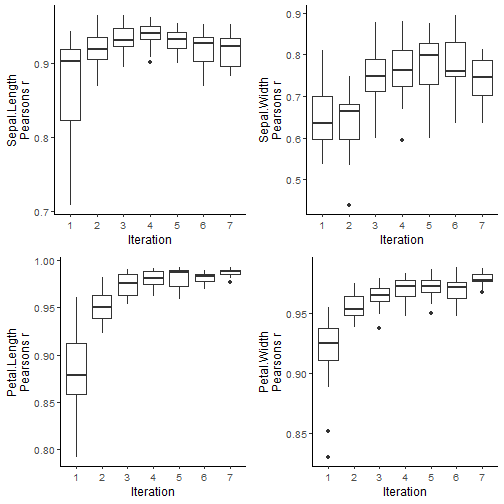Different correlation measures can be plotted by specifying `factCorrMetric` and `numbCorrMetric`.

### Center and Dispersion Convergence

Sometimes, if the missing data locations are correlated with higher or lower values, we need to run multiple iterations for the process to converge to the true theoretical mean (given the information that exists in the dataset). We can see if the imputed data converged, or if we need to run more iterations:

``````plotVarConvergence(miceObj,vars='allNumeric')
``````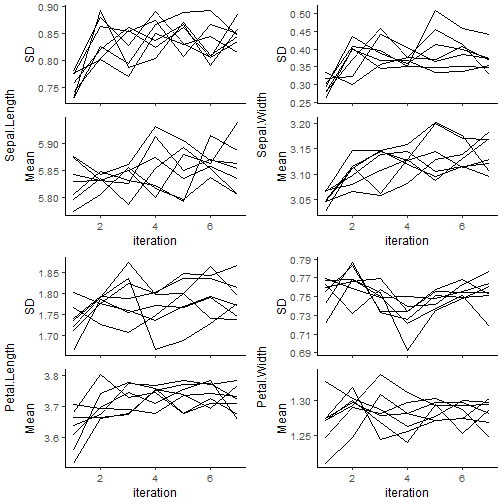It doesn't look like this dataset had a convergence issue. We wouldn't expect one, since we amputed the data above completely at random for each variable. When plotting categorical variables, the entropy will be plotted instead of the standard deviation, and the percentage of the mode will be plotted instead of the mean.

### Model OOB Error

Random Forests are nice because we have a cheap way to determine model error, without cross validation. Each model returns the OOB Accuracy, for classification, and r-squared, for regression. We can see how these converged as the iterations progress:

``````plotModelError(miceObj,vars='allNumeric')
``````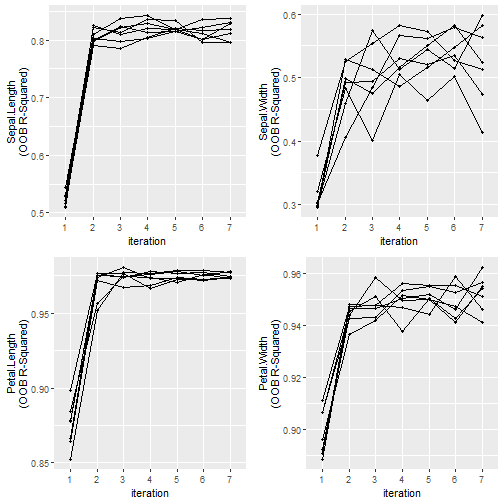It looks like the variables were imputed with a reasonable degree of accuracy. That spike after the first iteration was due to the nature of how the missing values are filled in before the models are run.

### Variable Importance

Now let's plot the variable importance for each imputed variable. The top axis contains the variable that was used to impute the variable on the left axis.

``````plotVarImportance(miceObj)
``````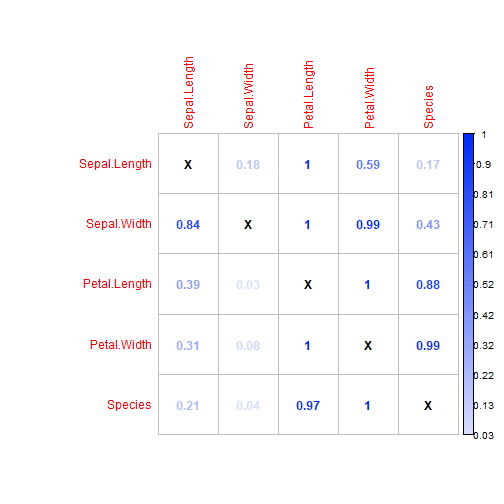The variable importance metric used is returned by ranger when `importance = 'impurity'`. Due to large possible variances in the returned value, the data plotted here has been 0-1 scaled within each imputed variable. Use `display = 'Absolute'` to show unscaled variable importance.

### Imputed Variance Between Datasets

We are probably interested in how “certain” we were of our imputations. We can get a feel for the variance experienced for each imputed value between the datasets by using `plotImputationVariance()` function:

``````plotImputationVariance(miceObj,ncol=1)
``````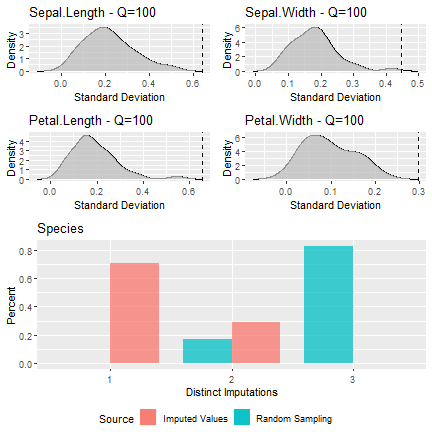When plotting the variance of categorical data, the distribution of the number of unique imputed levels is compared to the theoretical distribution of unique levels, given they were drawn randomly. You can see that most of the imputed values only had 1 imputed value across our 8 datasets, which means that the imputation process was fairly 'certain' of that imputed class. According to the graph, most of our samples would have had 3 different samples drawn, if they were drawn randomly for each dataset sample.
When plotting the variance of numeric features, the standard deviation of the imputed values is calculated for each sample. This is then compared to the total population standard deviation. Percentage of the samples with a SD below the population SD is shaded in the densities above, and the Quantile is shown in the title. The `iris` dataset tends to be full of correlation, so all of our imputations had a SD lower than the population SD, however this will not always be the case.

## Using the Imputed Data

To return the imputed data simply use the `completeData` function:

``````dataList <- completeData(miceObj)
head(dataList[],10)
``````
``````##     Sepal.Length Sepal.Width Petal.Length Petal.Width Species
##  1:          5.1         3.5          1.3         0.2  setosa
##  2:          4.9         3.0          1.4         0.2  setosa
##  3:          4.7         3.2          1.3         0.2  setosa
##  4:          4.6         3.1          1.5         0.2  setosa
##  5:          5.0         3.6          1.4         0.2  setosa
##  6:          5.4         3.9          1.7         0.4  setosa
##  7:          5.1         3.4          1.4         0.3  setosa
##  8:          5.0         3.4          1.5         0.2  setosa
##  9:          4.4         2.9          1.4         0.2  setosa
## 10:          4.9         3.1          1.5         0.1  setosa
``````

We can see how the imputed data compares to the original data before it was amputed: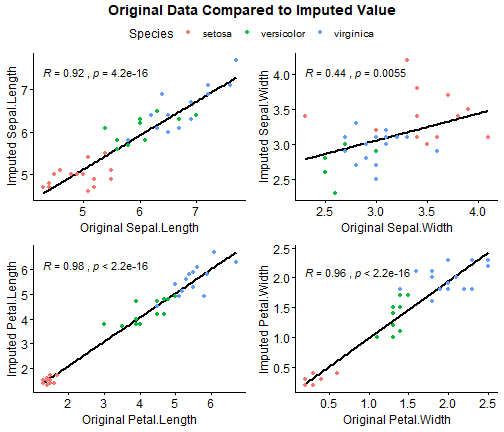It looks like most of our variables were imputed with a high degree of accuracy. Sepal.Width had a relatively poor Spearman correlation, however we expected this when we saw the results from `plotModelError()` above.